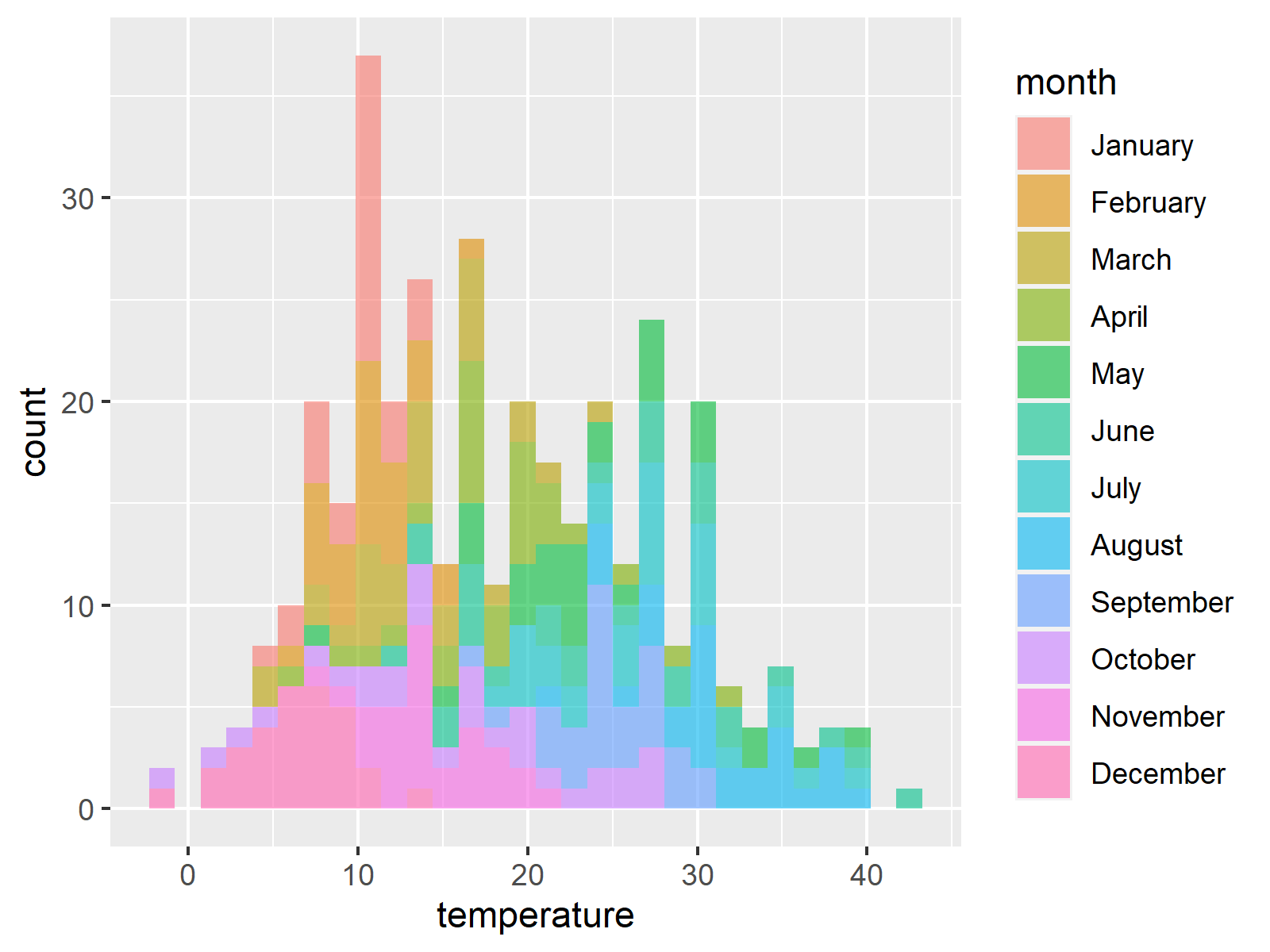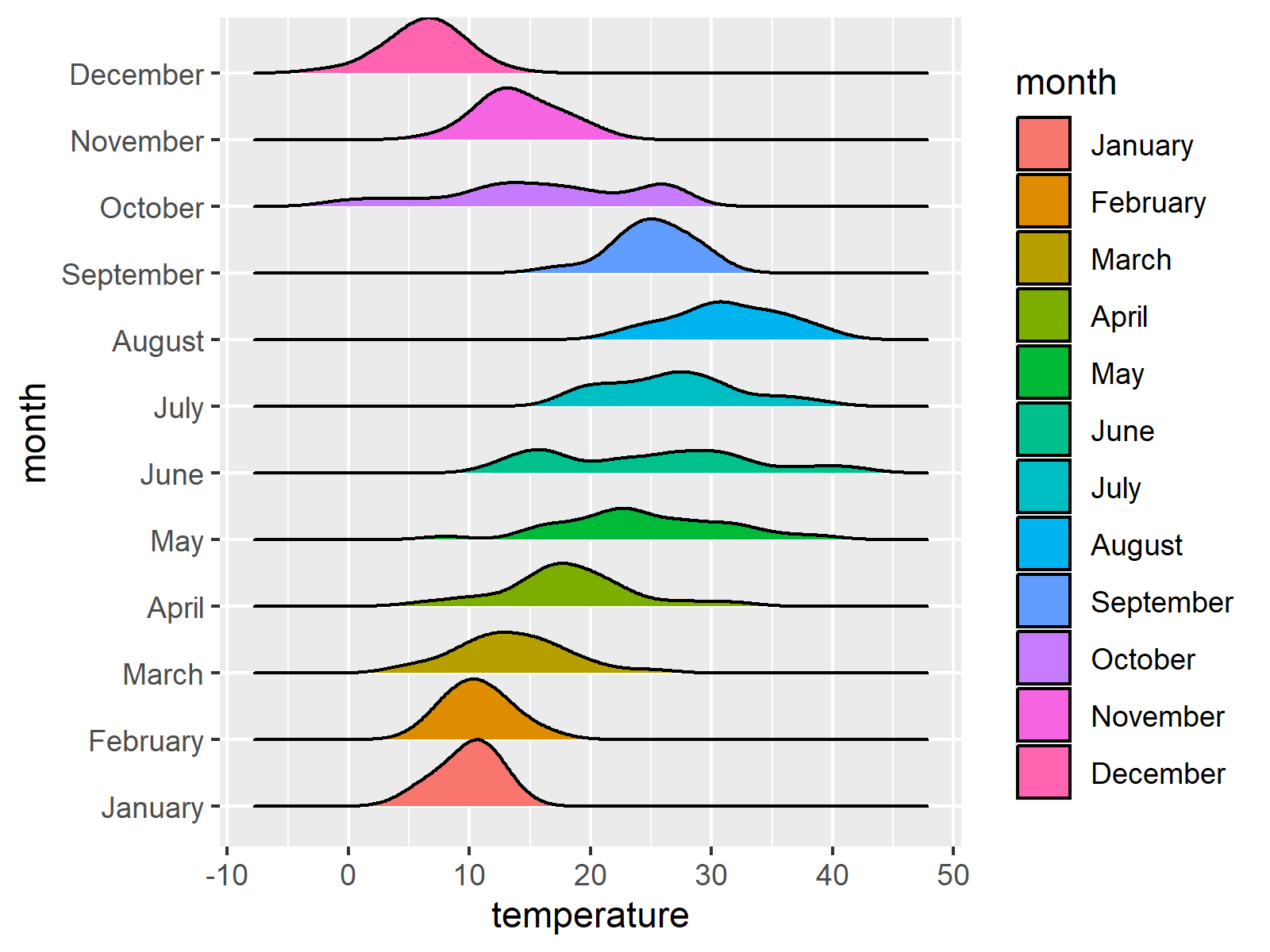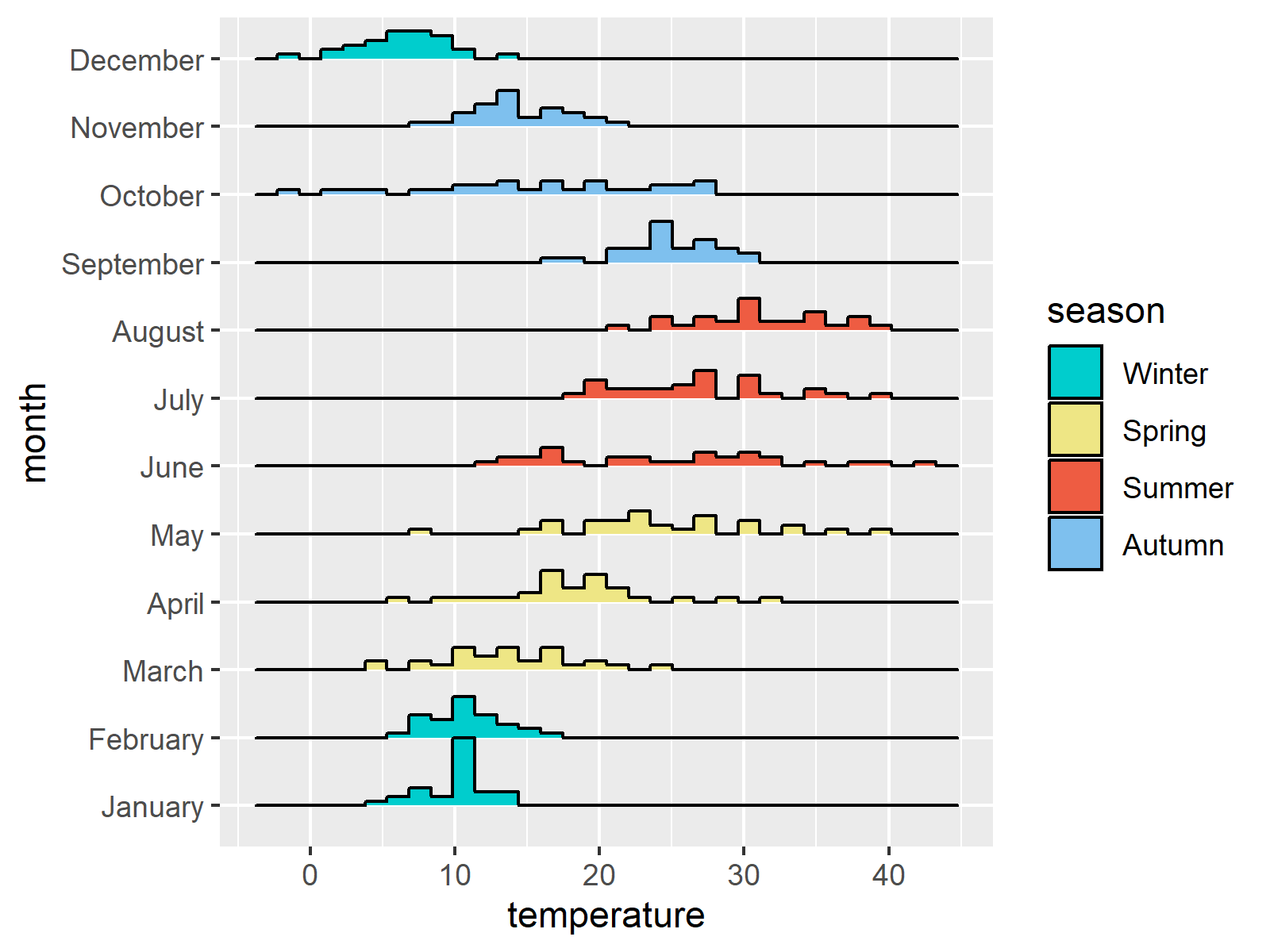# Ridgeline Plots in R (3 Examples)

This tutorial demonstrates how to create a ridgeline plot in the R programming language.

Let’s jump right to the examples!

## Example Data

In the first section of this tutorial, we’ll create some example data. For this, we have to install and load the tidyverse package:

```install.packages("tidyverse")                        # Install and load tidyverse
library("tidyverse")```

Next, we create a data frame containing temperatures for the different months of the year. We assume that each month has 30 days to facilitate the creation of the example.

Let’s generate the data:

```set.seed(1992)                                       # Set seed for reproducibility
example <- data.frame(day = 0, month = 0, temperature = 0)  # Create empty data

months_averages <- c(10, 11, 14, 18, 23, 26, 29, 32, 25 ,18, 14, 6)
months_sd <- c(3, 3, 4, 6, 7, 8, 5, 5, 4, 7, 4, 3)

for(i in 1:12) {                                     # Fill up data with random numbers
example[(i*30 - 29):(i*30), "day"] <-
1:30
example[(i*30 - 29):(i*30), "month"] <-
month.name[i]
example[(i*30 - 29):(i*30), "temperature"] <-
rnorm(n = 30,
mean = months_averages[i],
sd = months_sd[i]) %>%
round()
}

example\$month <-                                     # Convert month variable into a factor
example\$month %>%
as_factor()

#   day   month temperature
# 1   1 January          11
# 2   2 January          13
# 3   3 January          10
# 4   4 January           7
# 5   5 January          11
# 6   6 January           5```

The previous output of the RStudio console shows the structure of our example data. As you can see, our data contains the three columns day, month, and temperature.

Let’s draw these data!

## Alternatives to Ridgeline Plots

The typical alternative to ridgeline plots would be histograms. We could create an overlaid ggplot2 histogram of our example data using the following lines of code:

```histogram <-                                         # Draw histogram
example %>%
ggplot(aes( x = temperature, fill = month)) +
geom_histogram( alpha = 0.6)
histogram```Figure 1 shows the output of the previous R syntax. All the histograms were drawn together, what a mess!

Another alternative would be a facet plot where we facet against months to see every histogram in a separate panel:

```faceted_histogram <-                                 # Draw facet plot
histogram +
facet_wrap(~ month, nrow = 4)
faceted_histogram```Figure 2 shows our data in a facet plot. This already looks much better. However, an even cleaner way to visualize our data would be ridgeline plots.

We’ll draw such graphs in the next sections – so keep on reading!

## Example 1: Drawing Ridgeline Plot of Histograms

If we want to create ridgeline graphics in R, we first have to install and load the ggridges package:

```install.packages("ggridges")                         # Install and load ggridges package
library("ggridges")```

We can now use the geom_density_ridges function to draw the histograms in a ridgeline chart. In our plot, the x aesthetic will be the temperature. The y aesthetic will be the months, since we want to have each month separated.

```ridge_histogram_plot <-                              # Draw histograms in ridgeline plot
example %>%
ggplot(aes(x = temperature , y = month,  fill = month)) +
ggridges::geom_density_ridges(scale = 1,           # Overlap among histograms
stat = "binline")
ridge_histogram_plot```Figure 3 shows the output of the previous R code: We have created a ridgeline plot containing histograms.

## Example 2: Drawing Ridgeline Plot of Densities

We can also make density plots instead of histograms by removing stat = “binline” within the geom_density_ridges function:

```ridge_density_plot <-                                # Draw densities in ridgeline plot
example %>%
ggplot(aes(x = temperature , y = month,  fill = month)) +
ggridges::geom_density_ridges(scale = 1)           # Overlap among histograms
ridge_density_plot```As you can see in Figure 4, our histograms were replaced by densities.

## Example 3: Modifying the Groups in Ridgeline Plots

We can also use ridgeline charts to draw the same group (i.e. seasons instead of months) over several lines in our graphic.

For this, we first have to add another variable to our data, which converts our month variable into seasons:

```example\$season <-                          # Add seasons to better see the group of months
case_when(example\$month %in% month.name[c(12, 1, 2)] ~ "Winter",
example\$month %in% month.name[c(3:5)] ~ "Spring",
example\$month %in% month.name[c(6:8)] ~ "Summer",
example\$month %in% month.name[c(9:11)] ~ "Autumn" ) %>%
as_factor()
#   day   month temperature season
# 1   1 January          11 Winter
# 2   2 January          13 Winter
# 3   3 January          10 Winter
# 4   4 January           7 Winter
# 5   5 January          11 Winter
# 6   6 January           5 Winter```

In the next step, we can assign the colors and the legend labels of our plot depending on the month:

```ridge_histogram_by_season <-                         # Ridgeline plot with seasons
example %>%
ggplot(aes(x = temperature , y = month,  fill = season)) +
ggridges::geom_density_ridges(scale = 1,           # Overlap among histograms
stat = "binline") +  # Draw histograms
scale_fill_manual(values = c("cyan3",
"khaki2",
"tomato2",
"skyblue2"))
ridge_histogram_by_season```Figure 5 shows our final plot with colors corresponding to the seasons of the year. Note that the histograms shown in this ridgeline plot are the same as in Figure 3.

## Video & Further Resources

If you need further info on ridgeline plots, I recommend having a look at the following video on the Tech Know How YouTube channel:

Please accept YouTube cookies to play this video. By accepting you will be accessing content from YouTube, a service provided by an external third party.If you accept this notice, your choice will be saved and the page will refresh.

Also, you might want to read the other articles on the Statistics Globe website:

In summary: At this point of the tutorial you should know how to draw ridgeline plots in R. In case you have any further comments or questions, please write a comment below. Adrián and I look forward to hearing from you!

Subscribe to the Statistics Globe Newsletter

•Fernando Ramires
June 18, 2021 2:38 pm

on Example 2…
It is possible to create this map with 3 variables, month, days and temperature, and a color variation would reflect the temperature variation throughout each month????

•June 21, 2021 7:04 am

Regards

Joachim

•June 18, 2021 2:57 pm

Yes Fernando.

The histogram shows for each month(y-axis) the number of days(height of the histogram) for every temperature (x-axis).

If you want to show the temperature distribution along the month, just change fill aesthetic -> “fill = temperature”.

Hope this helps you !

•David
June 18, 2021 9:38 pm

This is really good. Another amazing tutorial from ‘Statistics Globe’, which has become my absolute reference. Thanks again Joachim! Excellent, as always.

•June 21, 2021 7:05 am

Thanks a lot for this amazing feedback David! I’m very glad to hear that you like Adrián’s and my work! 🙂

Regards

Joachim

•Azzeddine REGHAIS
June 19, 2021 6:03 am

Can I replace the temperature with precipitation in these examples?

•Azzeddine
June 19, 2021 6:51 am

In these examples, you used daily temperature data for one year
But in climatic studies, the average monthly temperature is usually used for a series consisting of several years (Ex: 1970-2020)
How is the temperature represented in this case?

•David
June 19, 2021 8:44 am

This is amazing! Once again, Statistics Globe is the best!
Thanks a lot Joachim! ¡Muchísimas gracias Adrián!
Kind Regards.

•June 21, 2021 7:08 am

Thanks again David, very glad to hear that you like our tutorial! 🙂

•June 21, 2021 8:48 am

For Fernando Ramires.

It is a bit tricky than replace “fill” aesthetic to change colors :

I let you the code to replace the fill color with temperature values:

example %>%
ggplot(aes(x = temperature , y = month, fill = ..x..)) +
scale_fill_viridis_c() # This line just change the colors, remove it if you want

•June 21, 2021 8:58 am

Azzeddine, that’s a good question.

Luckily, we have a good answer for that.

In this example, we have a used a small data.frame with just one year. We don’t even set that variable in the example data.frame.

So, if you have data from different years, you just will have more observations for each “month” and can represent the data in the exact same way (with histograms displayed in ridgeline plots).

If you want to compare the evolution of average temperature among years, you probably need a different chart (line-chart).

Answering to the question about precipitation. If you have precipitation data, you can substitute temperature by precipitation and your graph will be pretty similar 😉

•Azzeddine
June 21, 2021 11:14 am

I hope that another example will be added to clarify and explain this case (using data for several years)
My heartfelt greetings to you

•Azzeddine
June 22, 2021 5:36 am

I did what you told me
But I still get some problems like
The months are not in order
There is also the following message:

“`stat_bin()` using `bins = 30`. Pick better value with `binwidth`.

Can you explain sir?
Is it possible to contact you via e-mail to send you a copy of the code that you made, I think it would be better

•Azzeddine
June 22, 2021 5:41 am
•June 22, 2021 5:50 am

Hey Azzeddine,

The two problems you mentioned are not really related to ridgeline plot, but to ggplot2 in general.

You can reorder your legend as explained here: https://statisticsglobe.com/change-display-order-of-ggplot2-plot-legend-in-r

You can ignore the warning message, in case you are happy with the bin width.

I hope that helps!

Joachim

•Azzeddine
June 22, 2021 6:22 am

A very thanks Joachim
You know I’m a beginner in the field
Well, I’ll see what you suggested

Salutations

•Sonia
January 26, 2022 11:18 pm

Hi! First, congratulations! This is really my reference page for consulting!
Let me introduce you my problem. I have 3 variables: time point (0 to 14), a continuous variable (Z-score) and cell type. I would like to have a density plot for each cell type, on the X axis, the time points, and the height of each of the density plots would be the Z-score (one value for each timepoint). Is this possible?
ridge_histogram_plot % ggplot(aes(x = Day , y = Zscore, fill = Cluster)) + ggridges::geom_density_ridges(scale = 1)

Im trying this. Is it because the Zscore is not categorical?

Thanks so much!

Sonia

•February 7, 2022 11:42 am

Hey Sonia,

Thank you very much for the kind words, glad you find my tutorials helpful!

Excuse me for the delayed response, I took a few days off. Have you already found a solution for this?

Regards,
Joachim

•Kirby White
February 17, 2022 6:26 pm

Love it! Exactly the help I was looking for.

•February 18, 2022 9:05 am